# PCA Data PCA Data minus mean Eigenvectors Compressed

• Slides: 15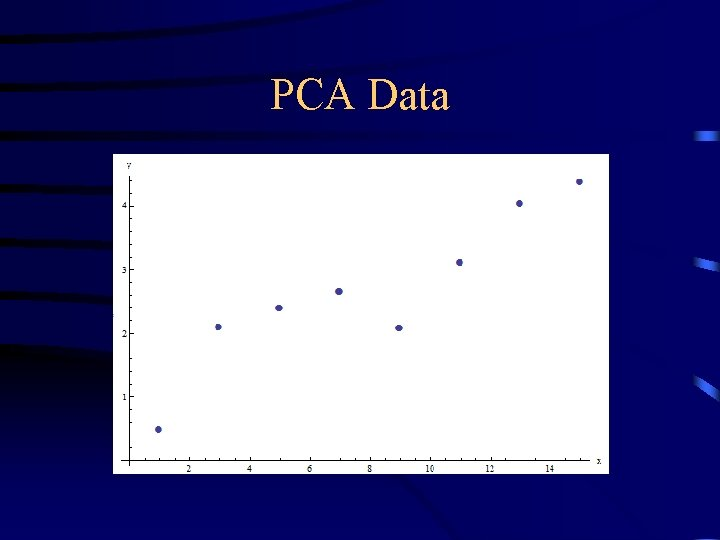PCA DataPCA Data minus mean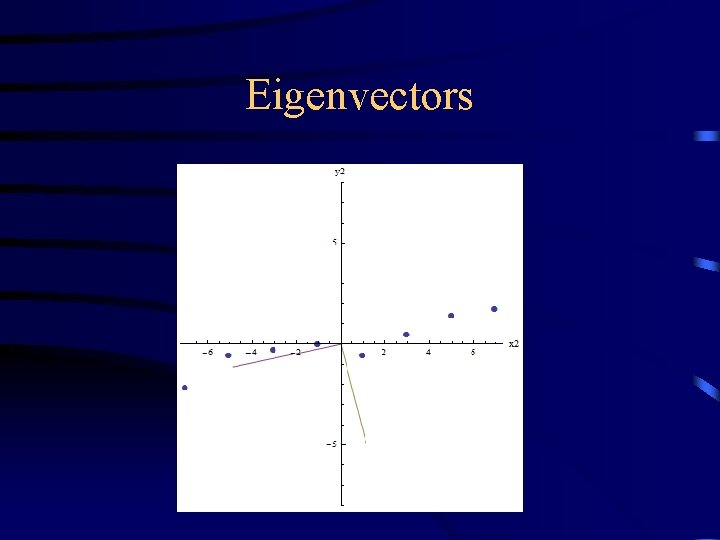EigenvectorsCompressed Data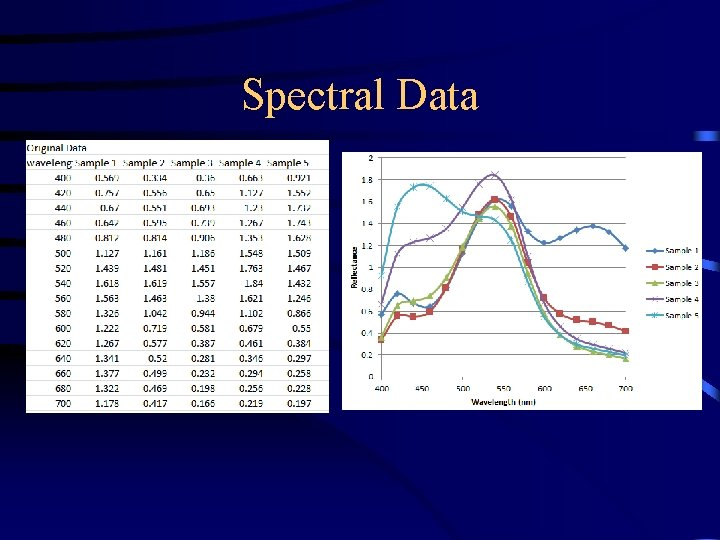Spectral Data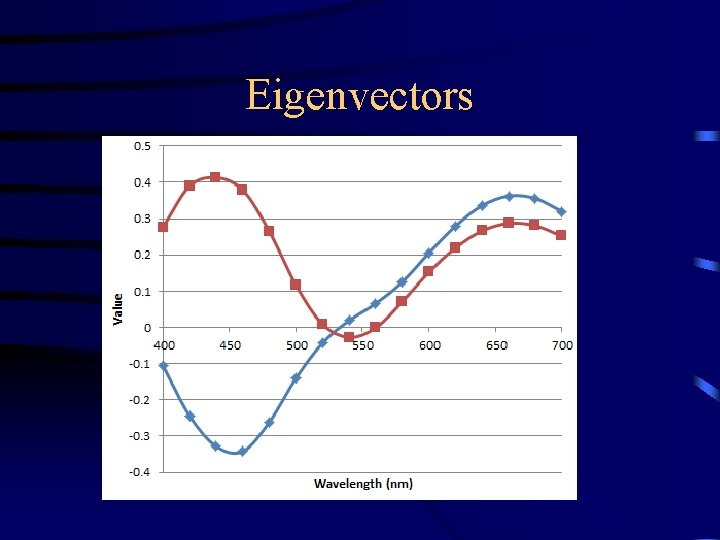Eigenvectors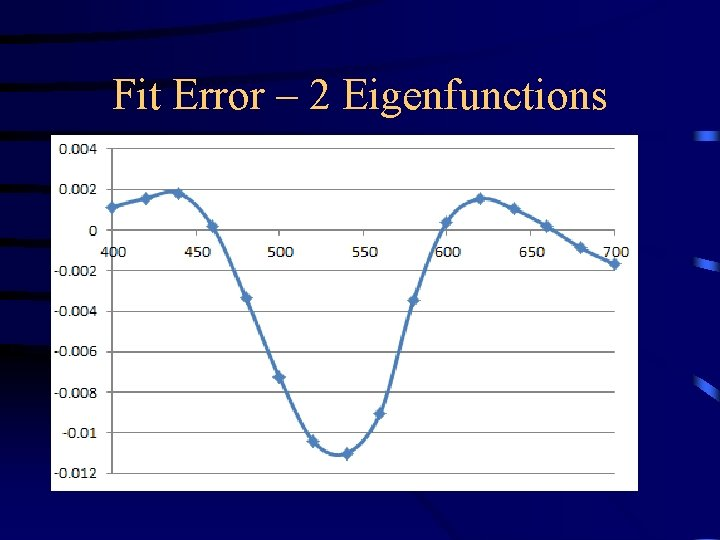Fit Error – 2 Eigenfunctions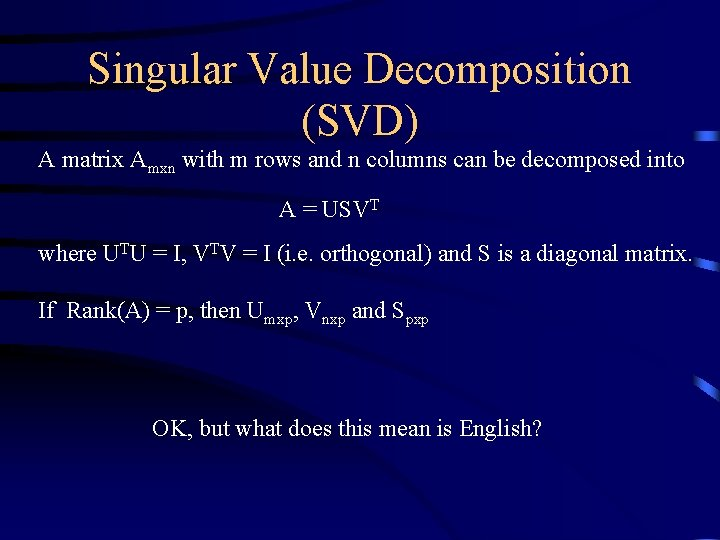Singular Value Decomposition (SVD) A matrix Amxn with m rows and n columns can be decomposed into A = USVT where UTU = I, VTV = I (i. e. orthogonal) and S is a diagonal matrix. If Rank(A) = p, then Umxp, Vnxp and Spxp OK, but what does this mean is English?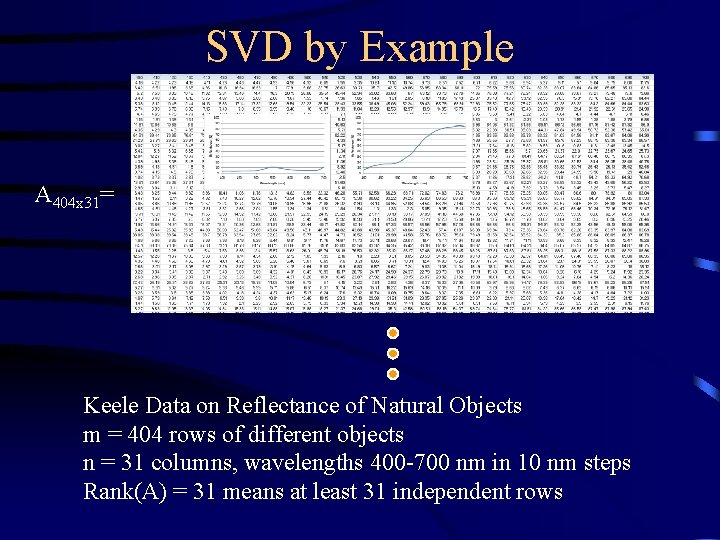SVD by Example A 404 x 31= Keele Data on Reflectance of Natural Objects m = 404 rows of different objects n = 31 columns, wavelengths 400 -700 nm in 10 nm steps Rank(A) = 31 means at least 31 independent rowsSVD by Example A 404 x 31 = U 404 x 31 S 31 x 31 VT 31 x 31 UTU = I means dot product of two different columns of U equals zero. VTV = I means dot product of two different columns of V (rows of VT) equals zero.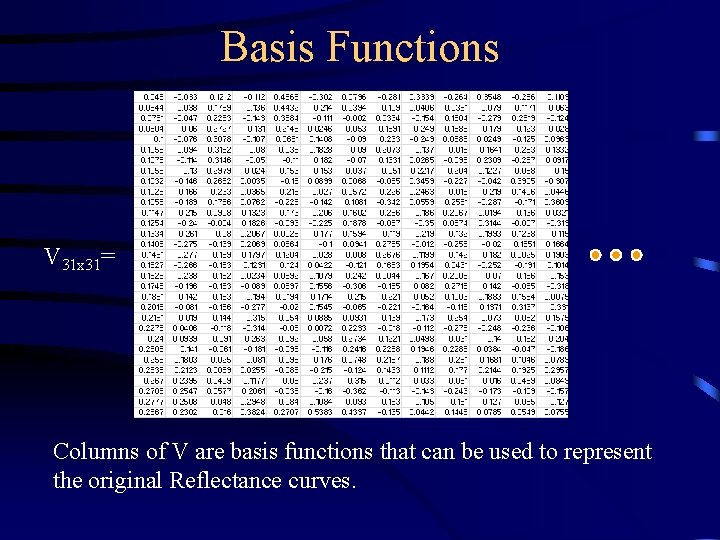Basis Functions V 31 x 31= Columns of V are basis functions that can be used to represent the original Reflectance curves.Basis Functions First column handles most of the variance, then the second column etc.Singular Values S 31 x 31= The square of diagonal elements of S describe the variance accounted for by each of the basis functions.SVD Approximation A 404 x 31 ~ U 404 xd Sdxd VTdx 31 The original matrix can be approximated by taking the first d columns of U, reducing S to a d x d matrix and using the first d rows of VT.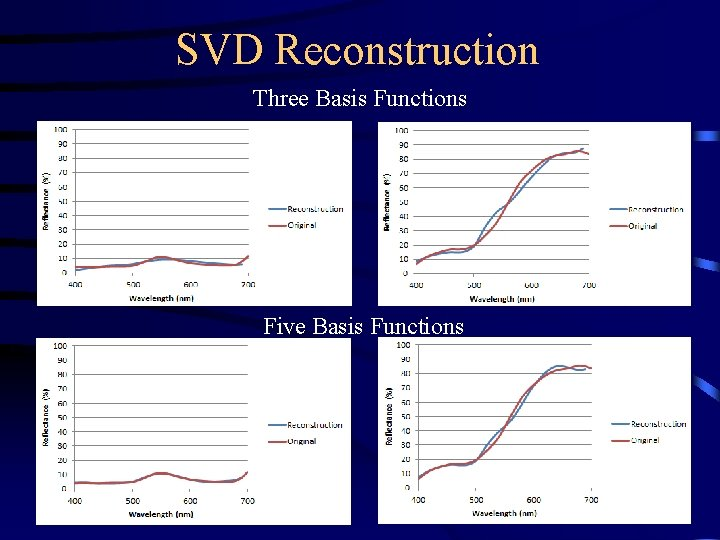SVD Reconstruction Three Basis Functions Five Basis Functions